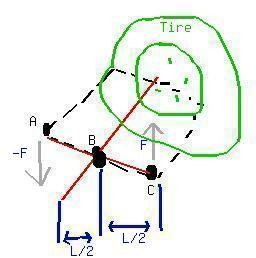Ask question

# Given f(x) cos(2x) and the interval [0, 3 frac{pi}{4}] approximate the area bounded by the graph of f(x) and the axis on the interval using a left, right, and mid point Riemann sum with n = 3# Given f(x) cos(2x) and the interval [0, 3 frac{pi}{4}] approximate the area bounded by the graph of f(x) and the axis on the interval using a left, right, and mid point Riemann sum with n = 3

Question
Confidence intervalsasked 2021-01-28
Given $$f(x)\ \cos(2x)$$ and the interval
$$[0,\ 3\ \frac{\pi}{4}]$$
approximate the area bounded by the graph of f(x) and the axis on the interval using a left, right, and mid point Riemann sum with $$n = 3$$

## Answers (1)2021-01-29

Step 1
Interval $$[0,\ 3\ \frac{\pi}{4}]$$ is to be devided into 3 sub-intervals whos length is given by
$$h=\ \frac{b\ -\ a}{n}=\frac{\frac{3\pi}{4}\ -\ 0}{3}=\frac{\pi}{4}$$
Sep 2
Therefore, the three sub-intervals are
$$\left[0,\ \frac{\pi}{4}\right],\ \left[\frac{\pi}{4},\ \frac{2\pi}{4}\right]\left[\frac{2\pi}{4},\ \frac{3\pi}{4}\right]$$
Step 3
Area bounded by the graph of f(x) and the axis on the interval using a left end point Riemann sum id given by
$$A_{L}=h\left[|f(0)|\ +\ |f\left(\frac{\pi}{4}\right)|\ +\ |f\left(\frac{2\pi}{4}\right)|\right]$$
$$=\frac{\pi}{4}\left[|\cos\ 0|\ +\ |\frac{\cos\ \pi}{2}|\ +\ |\cos\ \pi|\right]=\frac{\pi}{4}[1\ +\ 0\ +\ 1]=\frac{\pi}{2}$$
Step 4
Area bounded by the graph of f(x) and the axis on the interval using a right end point Riemann sum is given by
$$A_{r}=h\left[|f\left(\frac{\pi}{4}\right)|\ +\ |f\left(\frac{2\pi}{4}\right)|\ +\ |f\left(\frac{3\pi}{4}\right)|\right]$$
$$=\frac{\pi}{4}\left[|\cos\ \times\ \frac{\pi}{2}|\ +\ |\cos\ \pi|\ +\ |\cos\ \times\ \frac{3\pi}{2}|\right]=\frac{\pi}{4}[0\ +\ 1\ +\ 0]=\frac{\pi}{4}$$
Step 5
Area bounded by the graph of f(x) and the axis on the interval using a mid point Riemann sum is given by
$$A_{m}=\left[\left|f\left(\frac{\pi}{8}\right)\right|\ +\ \left|f\left(\frac{3\pi}{8}\right)\right|\ +\ \left|f\left(\frac{5\pi}{8}\right)\right|\right]$$
$$=\frac{\pi}{4}\left[\left|\cos\ \times\ \frac{\pi}{4}\right|\ +\ \left|\cos\ \times\ \frac{3\pi}{4}\right|\ +\ \left|\cos\ \times\ \frac{5\pi}{4}\right|\right]=\frac{\pi}{4}\left[\frac{1}{\sqrt{2}}\ +\ \frac{1}{\sqrt{2}}\ +\ \frac{1}{\sqrt{2}}\right]=\frac{3\pi}{4\sqrt{2}}$$

### Relevant Questionsasked 2021-02-26
Consider the function $$f(x) = \sin x$$ on th interval [0, 3]. Let P be a uniform partition of [0, 3] with 4 sub-intervals. Compute the left and right Riemann sum of f on the partition. Enter approximate values, rounded to three decimal places.asked 2020-11-14
Consider the following.
$$f(x) = 49 - x^{2}$$
from $$x = 1 to x = 7, 4$$ subintervals
(a) Approximate the area under the curve over the specified interval by using the indicated number of subintervals (or rectangles) and evaluating the function at the right-hand endpoints of the subintervals.
(b) Approximate the area under the curve by evaluating the function at the left-hand endpoints of the subintervals.asked 2021-01-31
Consider the function $$f(x) = x^{2}$$ on the interval [1, 9]. Let P be a uniform partition of [1,9] with 16 sub-intervals. Compute the left and right Riemann sum of f on the partition. Use exact values.asked 2021-02-26

The reason ehy the point $$(-1, \frac{3\pi}{2})$$ lies on the polar graph $$r=1+\cos \theta$$ even though it does not satisfy the equation.asked 2021-06-05
Two random variables X and Y with joint density function given by:
$$f(x,y)=\begin{cases}\frac{1}{3}(2x+4y)& 0\leq x,\leq 1\\0 & elsewhere\end{cases}$$
Find the marginal density of X.asked 2021-05-08
Two random variables X and Y with joint density function given by:
$$f(x,y)=\begin{cases}\frac{1}{3}(2x+4y)& 0\leq x,\leq 1\\0 & elsewhere\end{cases}$$
Find the marginal density of Y.asked 2021-06-07
Find the absolute maximum and absolute minimum values of f on the given interval.
$$f(x)=5+54x-2x^{3}, [0,4]$$asked 2021-01-24
Suppose that the n-th Riemann sum (with n sub-intervals of equal length), for some function f(x) on the intrval [0, 2], is $$S_{n} = \frac{2}{n^{2}}\ \times\ \sum_{k = 1}^{n}\ k.$$
What is the value of $$\int_{0}^{2}\ f(x)\ dx?$$asked 2021-05-18
Two random variables X and Y with joint density function given by:
$$f(x,y)=\begin{cases}\frac{1}{3}(2x+4y)& 0\leq x,\leq 1\\0 & elsewhere\end{cases}$$
Find $$P(x<\frac{1}{3})$$asked 2021-03-26
A pair of forces with equal magnitudes, opposite directions,and different lines of action is called a "couple". When acouple acts on a rigid object, the couple produces a torque thatdoes not depend on the location of the axis. The drawing shows acouple acting on a tire wrench, each force being perpendicular tothe wrench. Determine an expression for the torque produced by thecouple when the axis is perpendicular to the tired and passesthrough (a) point A, (b) point B, and (c) point C. Express youranswers in terms of the magnitude F of the force and the length Lof the wrench...